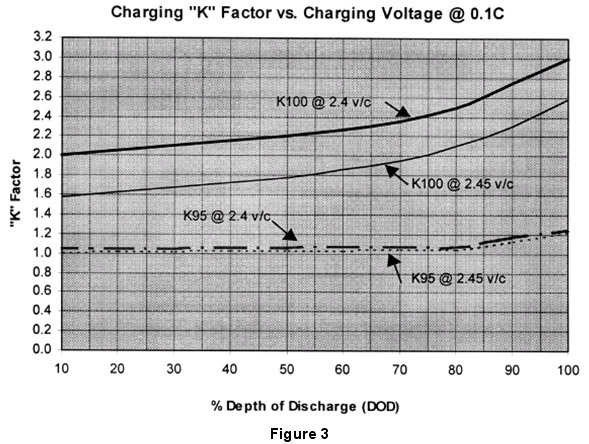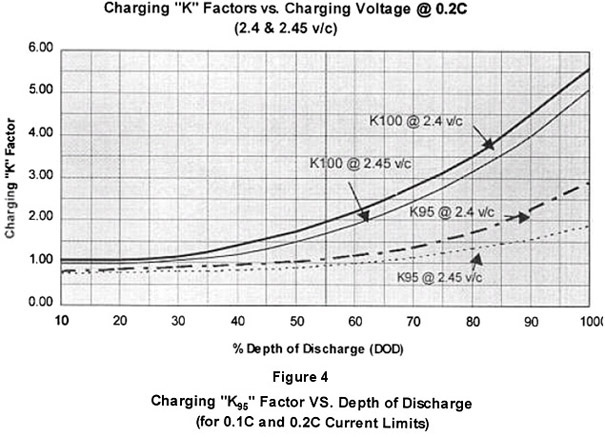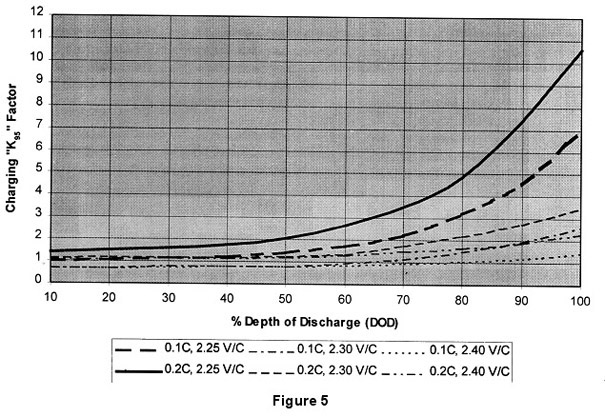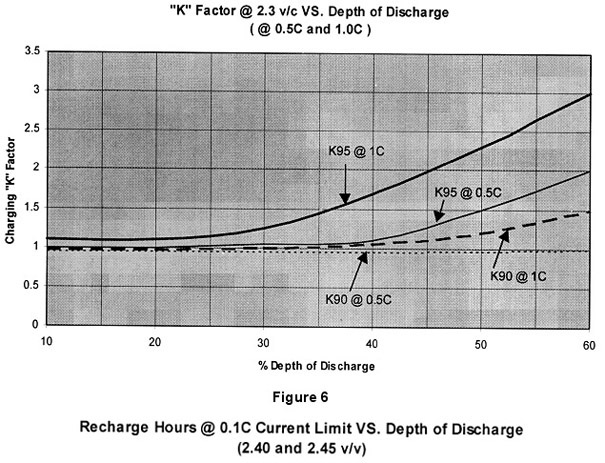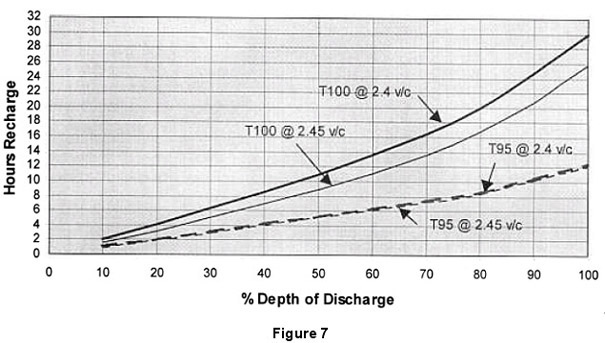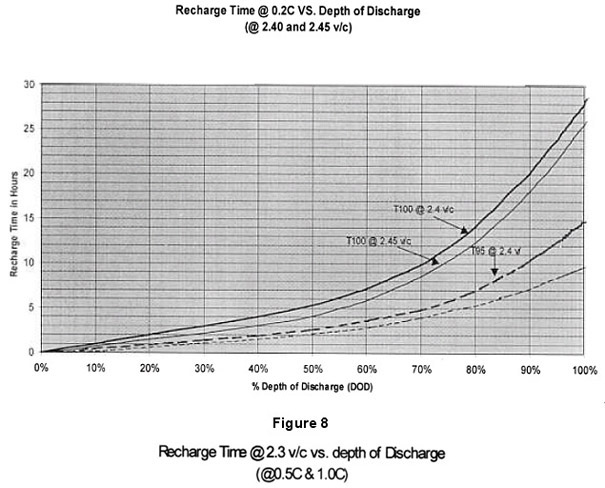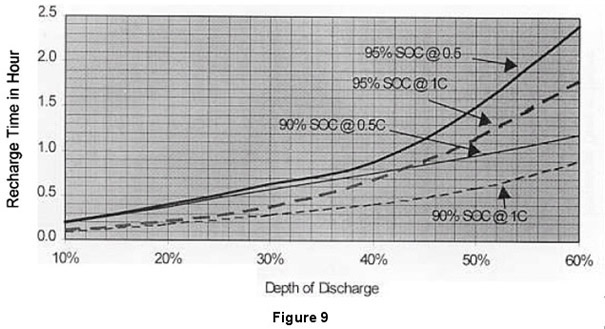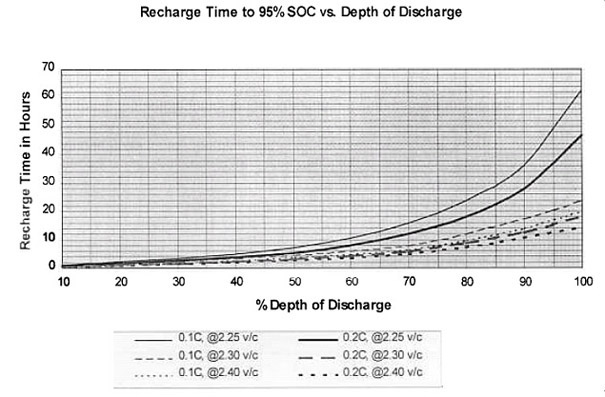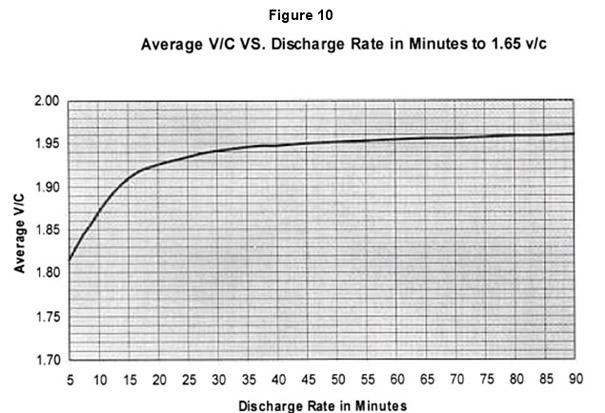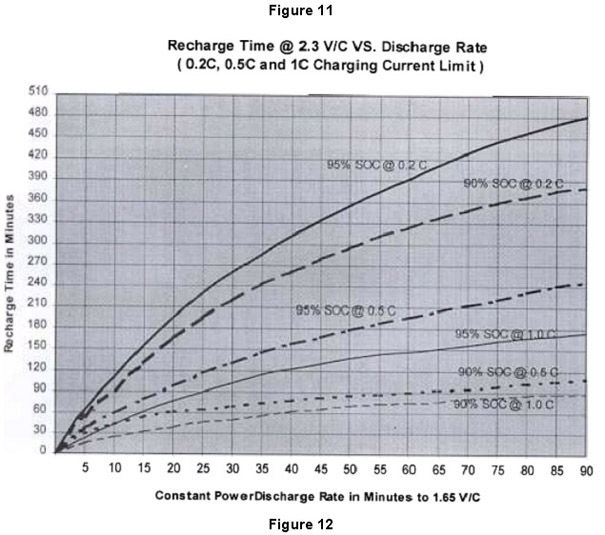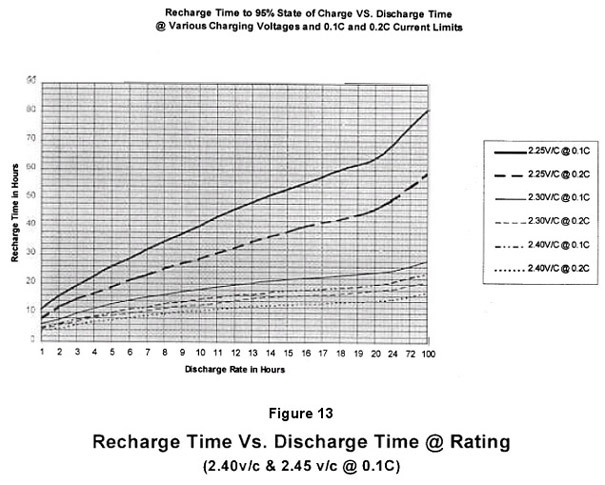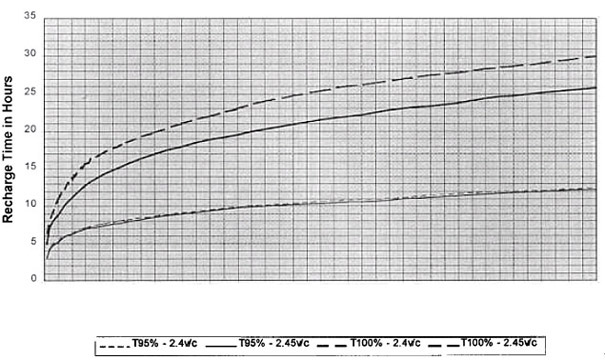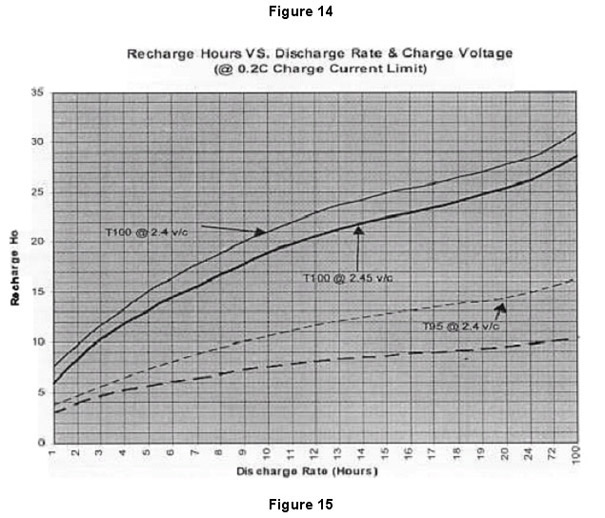Determining the time required to recharge a battery can be a difficult problem because it varies with depth of discharge (DOD), rate of discharge, charging current available (Ic, charging voltage and the state of charge (SOC) to which the battery is to be restored. To fully recharge the battery it is required to replace between 107% and 110% of the Ah removed during the discharge. However, if the battery is sized to provide 110% of the required capacity, it is only necessary to recharge the battery to a 90% SOC before the required autonomy can again be supplied. Likewise, if the battery is sized to provide 105% of the required capacity, it is only necessary to recharge the battery to a 95% SOC before the battery can again supply the required autonomy. This concept is important to understand in that under certain conditions it takes approximately twice the time to attain a 100% SOC as it does to attain 95% and four times as long as it does to attain 90% as is noted in Figure 1.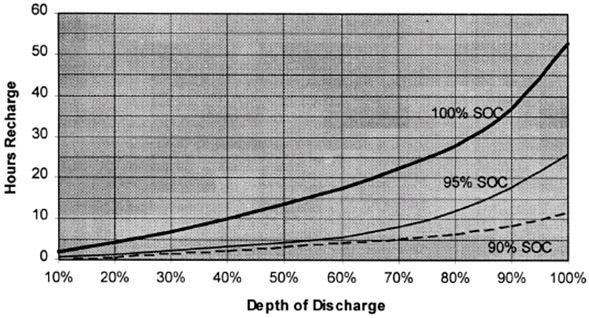Figure 1 - Recharge Time vs. Depth of Discharge A profile of battery current acceptance and charger output voltage during a typical recharge is illustrated in Figure 2. This profile will vary depending on the depth of the preceding discharge and the charger output characteristics. In general however, that period known as the "bulk phase" takes the battery to approximately an 85% to 90% SOC while the so called "absorption phase", with declining current acceptance, takes the battery to a 95% SOC. The last 5% of capacity is restored during what is called the "float phase". Due to the very low current acceptance during the "float phase" an extensive period of time is required to attain the necessary ampere hours of recharge to reach a 100% SOC. The highest recharging efficiency (approximately 98%) is attained during the "bulk phase of the recharge while the efficiency diminishes during the "absorption" and "float" phases to about 10% or less. Attainment of 100% SOC therefor requires an excessive amount of time due to the low current acceptance and poor efficiency during the float phase. Typically it is most practical to oversize the battery by 5 to 10 % to assure the battery can deliver the required autonomy at a 95% or 90% SOC following a reasonable recharge period as shown in Figure 1.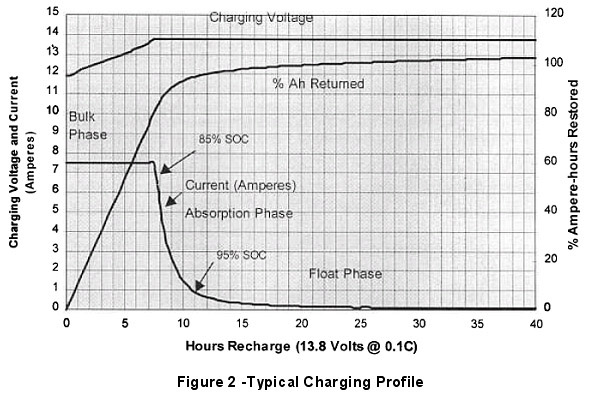RECHARGE TIME DETERMINATION Equation 1 can be used to determine the approximate time required to recharge the battery to a given state of charge (SOC) utilizing the appropriate " KX” factor from the curves in Figures 3, 4, 5 & 6.  These "KX" factors have been determined empirically and consider discharge rate, depth of discharge (DOD), charging current (IC) available and charging voltage. The following table provides some guidance on what are thought to be practical limits as a function of the system voltage.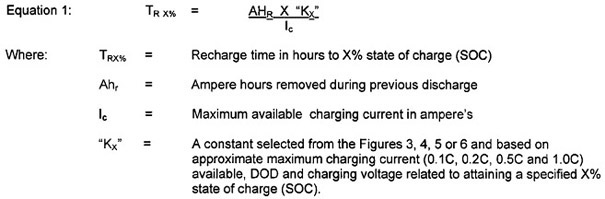Portable Power (Cycle Service) Recharge Time Determination Assume that a 65 Ah (C20) battery has been discharged at 5.85 ampere rate for 10 hours and that it is to be recharged to 100% SOC with a -/-ampere (Ic) charger having an output of 14.4 VDC.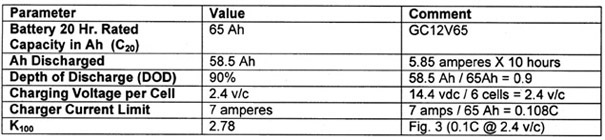Referring to Figure 3, the K100 value for 2.40 v/c at 0.1C is determined as 2.78. The recharge time to a 100% SOC is then calculated as: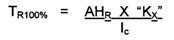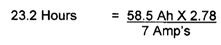This can also be verified utilizing the Recharge Time vs. DOD" curves of Figure 7 as approximately 24 hours. Assuming that the user could not wait for the required 100% SOC to be completed, the 95% SOC time requirement could be determined utilizing the Kg, factor of 1.2 as found in Figure 3.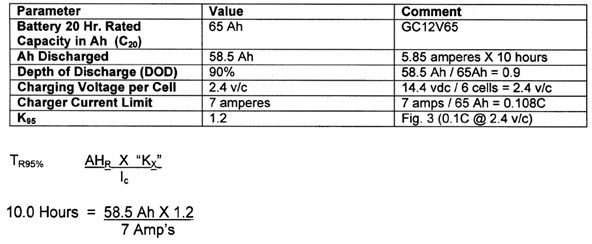Again, referring to Figure 7 a recharge time from 90% DOD is noted as approximately 10 hours When reviewing Figure 7 it will be noted that there is very little advantage to using 2.45 v/c rather than 2.40 v/c when the recharge is to only a 95% SOC using a 0.1C current limit. Under these same current limit conditions, the time savings to 100% SOC is only 10 to 15% through use of the higher charging voltage. However, as noted in Figure 8, when using a current limit of 0.2C the recharge time savings can be as much as 30% to a 95% SOC but less than 10% to a 100% SOC. Further, when comparing Figures 7 and 8 it will be noted that while use of 0.2C current limit (rather than 0.1C) can provide as much as a 20% reduction in recharge time to 95% SOC, the reduction in recharge time to 100% SOC is only approximately 5%. As can be seen, there is marginal advantage to use of the higher (2.45 v/c) charging voltage and current availability (0.2C) when charging to 100% SOC. However a significant recharge time advantage of up to 60% is realized if the battery is only required to be recharged to 95% SOC. If the battery is repeatedly recharged to only a 95% SOC it could result in it "cycling down"; that is, each successive discharge would produce less capacity. To prevent it from "cycling down" the battery should occasionally be recharged for 120% of the Kl00 time (e.g. 23 hours X 1.2 = 27.6 hours) to assure all residual lead sulfate in the plates is occasionally converted to the original active materials. Telecommunications Service Battery Recharge Time Determination In telecommunications applications the battery is typically sized to provide 3, 5 or 8 hours of autonomy. This results in typical depths of discharge of 75%, 81 % and 88% (relative to the C20 rating) respectively as noted in Table 1.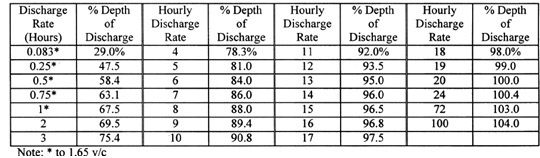Table 1- Discharge Rate VS. Depth of Discharge The typical charging voltage is 2.25 or 2.30 volts per cell (54.0 or 55.2 vdc respectively for a 48-volt system). Assume a GB 6FM100G (12V100/20Hr) telecommunications battery (100 Ah @ 20 hour rate) had been discharged at the 5-hour rate of 16.2 amperes to 1.75 v/c. Further assume the available charging current was 20 amperes and the charging voltage was 13.8 volts per 12-volt block.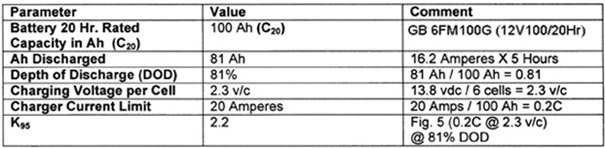Since the available charging current is 20 amperes or 0.2C (20 amperes per 100 Ah rated capacity), the “K95” factor would be determined as 2.2 using intersection of the 81% DOD line with the "0.2C @ 2.3 v/c" curve of Figure 5. The time then required to attain a 95% SOC following the 5-hour rate discharge would be: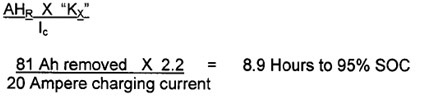It is also shown as approximately 9 hours in the recharge time curves of Figure 10 for an 81% depth of discharge. If the charging voltage utilized were 13.5 vdc ( 2.25 v/c) the recharge time to 95% SOC would be considerably longer as noted in the following example.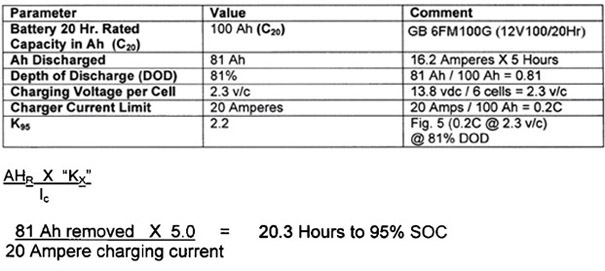This recharge time requirement can be confirmed as approximately 20 hours in Figure 10 In reviewing Figure 10 it will be noted that by simply using 2.30 v/c rather than 2.25 v/c at 0.2C current limit, there can be as much as a 60% reduction in the recharge time to 95% SOC. However, when 0.2C rather than 0.1C current limit is utilized at 2.3 v/c there is only approximately a 25% reduction in recharge time to 95% SOC. This leads to the conclusion that use of the higher charging voltage (2.30 v/c) is a more cost effective method to reduce recharge time than use of the higher current availability (I, = 0.2C). UPS Service Recharge Time Determination UPS systems place a constant power load on the battery system. That is, as the battery voltage declines during discharge the current drawn from the battery will increase such that the product of the battery voltage and current will always equal a constant wattage( P=V X 1 ). Therefore to determine the Ah removed during a discharge it is necessary to determine the approximate average current and voltage over the duration of the discharge. The approximate average current can be determined by dividing the watts I cell provided by the approximate average voltage per cell for the discharge period as shown in curve of Figure 11. Summary Recharge time is most cost effectively reduced when the battery is oversized by 5% or 10% thus requiring only a 95% or 90% SOC to provide the required autonomy. When up to 24 hours can be allowed for recharging it can most cost effectively be done at 2.40 v/c at a current availability of 0.1 C (1 0 amperes per 100 Ah). Typically, recharge time can be most cost effectively reduced, by up to 60%, by simply using 2.30 v/c charging voltage rather than 2.25 v/c. Recharge time is a function of rate and depth of the preceding discharge, recharge voltage, available charging current, desired state of charge and temperature. To determine the anticipated recharge time at 77OF, the following equation can be used.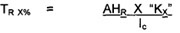The "Kx is a variable determined through testing as a function of depth of discharge (DOD), charging current availability (Ic), charging voltage and final state of charge (% SOC) and are presented in Figures 3, 4, 5 and 6. To calculate ampere-hours removed during a constant current discharge, simply multiply the ampere load by the duration of the load in hours ( AHR = Load Amperes * Hours Duration). When the ampere-hours removed (AHR) must be calculated for a constant power load it can be calculated as: "X" Ah, = (watts per cell I average v/c) X Hours discharge duration The following charts can be used in either the sizing of charger current limits and the determination of recharge times based on charging voltage, charging current limitation, depth of discharge or discharge time at rating. They are grouped as follows: " K Factor vs. Depth of Discharge - Figures 3, 4, 5 and 6 Recharge Time vs. Depth of Discharge - Figures 7, 8, 9 and 10 Recharge Time vs. Discharge Time @ Rating - Figures 11, 12, 13 and 14.